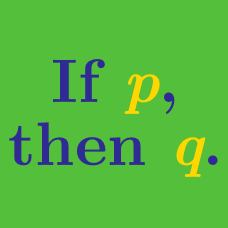Everyday Math

# Logic

Several friends are planning a road trip. If John goes on the road trip, then Kelly goes on the road trip. If Kelly does not go on the road trip, then Lawrence does not go on the road trip. Which of the following statements must be true?

(A)$\ \$ If John goes on the road trip, then Lawrence goes on the road trip.
(B)$\ \$ If John does not go on the road trip, then Kelly does not go on the road trip.
(C)$\ \$ If John does not go on the road trip, then Lawrence goes on the road trip.
(D)$\ \$ If Lawrence goes on the road trip, then John goes on the road trip.
(E)$\ \$ If Lawrence goes on the road trip, then Kelly goes on the road trip.

If Danny owns at least $4$ bikes, then Edward owns $3$ bikes. If Edward owns a bike, then Freddy owns $5$ bikes. If Danny owns $5$ bikes, which of the following statements must be true?

$\begin{array}{r r l} &\text{I.} & \text{Edward owns at least one bike.}\\ &\text{II.} &\text{Freddy owns at least one bike.}\\ &\text{III.} & \text{Freddy does not own any bike.}\\ \end{array}$

(A)$\ \$ I only
(B)$\ \$ II only
(C)$\ \$ III only
(D)$\ \$ I and II only
(E)$\ \$ I and III only

If Aria went to the school play, then Barney also went to the school play.
If Barney went to the school play, then Carie also went to the school play.
If Barney did NOT go to the school play, which of the following must be true?

$\begin{array}{r r l} &\text{I.} &\text{Aria went to the school play.}\\ &\text{II.} & \text{Carie went to the school play.}\\ &\text{III.} & \text{Aria did not go to the school play.}\\ \end{array}$

(A)$\ \$ I only
(B)$\ \$ III only
(C)$\ \$ I and II only
(D)$\ \$ II and III only
(E)$\ \$ None of the statements

If John calls May in class, May looks back. If Matthew pricks John in class, John calls May. If John did NOT call May in class, which of the following statements must be true?

$\begin{array}{r r l} &\text{I.} &\text{Matthew did not prick John.}\\ &\text{II.} & \text{May did not look back.}\\ &\text{III.} & \text{Matthew pricked John.}\\ \end{array}$

(A)$\ \$ I only
(B)$\ \$ III only
(C)$\ \$ I and II only
(D)$\ \$ II and III only
(E)$\ \$ None of I, II or III

The union of sets $X$ and $Y$ is the set $Z$. Which of the following statements must be true?

$\begin{array}{r r l} &\text{I.} & \text{If}\ 5\ \text{is in}\ X,\ \text{then}\ 5\ \text{is in}\ Y.\\ &\text{II.} & \text{If}\ 6\ \text{is in}\ Y,\ \text{then}\ 6\ \text{is in}\ Z.\\ &\text{III.} & \text{If}\ 7\ \text{is in}\ Z, \text{then}\ 7\ \text{is in}\ X.\\ \end{array}$

(A)$\ \$ I only
(B)$\ \$ II only
(C)$\ \$ III only
(D)$\ \$ II and III only
(E)$\ \$ I, II, and III

×